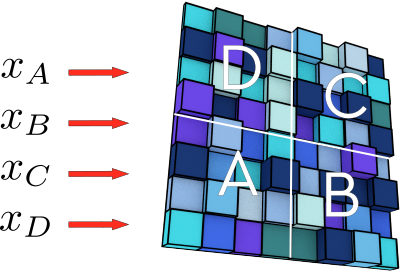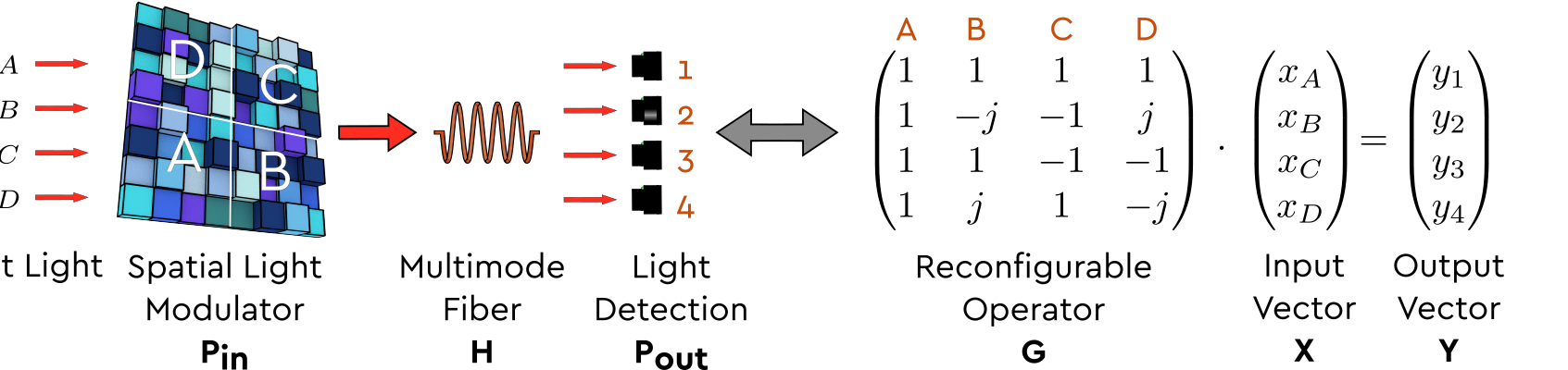## Wavefront shaping in complex media for analog computation[M. W. Matthès et al., Optica, 6 (2019)]

Performing linear operations using optical devices is a crucial building block in many fields ranging from telecommunications to optical analog computation and machine learning. For many of these applications, key requirements are robustness to fabrication inaccuracies, reconfigurability, and scalability. Traditionally, the conformation or the structure of the medium is optimized in order to perform a given desired operation. Since the advent of wavefront shaping, we know that the complexity of a given operation can be shifted toward the engineering of the wavefront, allowing, for example, to use any random medium as a lens.

In [M. W. Matthès et al., Optica, 6 (2019)], we propose to use this approach to use complex optical media such as multimode fibers or scattering media as a computational platform driven by wavefront shaping to perform analog linear operations. Given a large random transmission matrix representing the light propagation in such a medium, we can extract any desired smaller linear operator by finding suitable input and output projectors. We demonstrate this concept by finding input wavefronts using a Spatial Light Modulator that causes the complex medium to act as a desired complex-valued linear operator on the optical field.

The method we propose relies on the knowledge of the transmission matrix $$\mathbf{H}$$ of the complex medium. If one controls $$N$$ channels entering the medium and measures $$M$$ output channels, The transmission matrix is a $$M \times N$$ seemingly random complex matrix that links the output field $$\mathbf{Y}$$ to the input one $$\mathbf{X}$$ with $$\mathbf{Y} = \mathbf{H}\mathbf{X}$$.To create a system performing a linear operation represented by the matrix $$\mathbf{G}$$ of size $$m \times n$$ with $$m<M$$ and $$n<N$$, we identify adequate input and output projections $$\mathbf{P_{in}}$$ and $$\mathbf{P_{out}}$$ that satisfy

$$\mathbf{G} = \mathbf{P_{out}^{T}}\mathbf{H}\mathbf{P_{in}}$$Figure 1. Schematic representation of the method.

We present an implementation of our method which uses a fast digital micro-mirror device (DMD) and relies only on intensity measurements. We present the key elements of our method in Fig. 1 as well as the mathematical calculation it performs. After calculating the optimal input projection, the system is then ready to act as the desired linear operator $$\mathbf{G}$$.  The operation is performed by encoding the input signals $$\mathbf{X} = (x_1, x_2, ..., x_n)^T$$ onto as many incoming light beams directed towards the DMD, and then collecting the output signals on a camera.

Figure 2. Experimental results with a multimode fiber.

To illustrate the capability of our scheme, we present an implementation of a discrete Fourier transform operator of size $$16 \times 16$$ in Fig 2. We quantify the quality of the operation by estimating the operator's fidelity $$F_c = Tr(|\widetilde{\mathbf{G}}.\mathbf{G}^ \dagger |^2)*n$$, with $$\widetilde{\mathbf{G}}$$ the response matrix of our physical system. We also measure the Pearson correlation coefficient between experimental and predicted outputs. We present in Fig 2. the results. We obtain a correlation of $$0.912 \pm 0.027$$ and a fidelity of $$0.792 \pm 0.027$$. A good agreement between the experimental results and the ideal operation outputs is observed without averaging. In the paper, we show qualitatively similar results when using a scattering medium instead of a multimode fiber.

These results demonstrate the possibility of performing reliable one-shot linear operations through a MMF and scattering media. Switching between different operators can be done at will, the only requirement is to reconfigure the SLM to display the projection corresponding to the new operator. We show in [M. W. Matthès et al., Optica, 6 (2019)] that this approach can be scaled up to perform operations of rank $$n\approx250$$ in MMF and $$n\approx1000$$ using scattering media, which would allow envisioning the implementation of optical artificial neural networks.

Created by sebastien.popoff on 15/04/2019